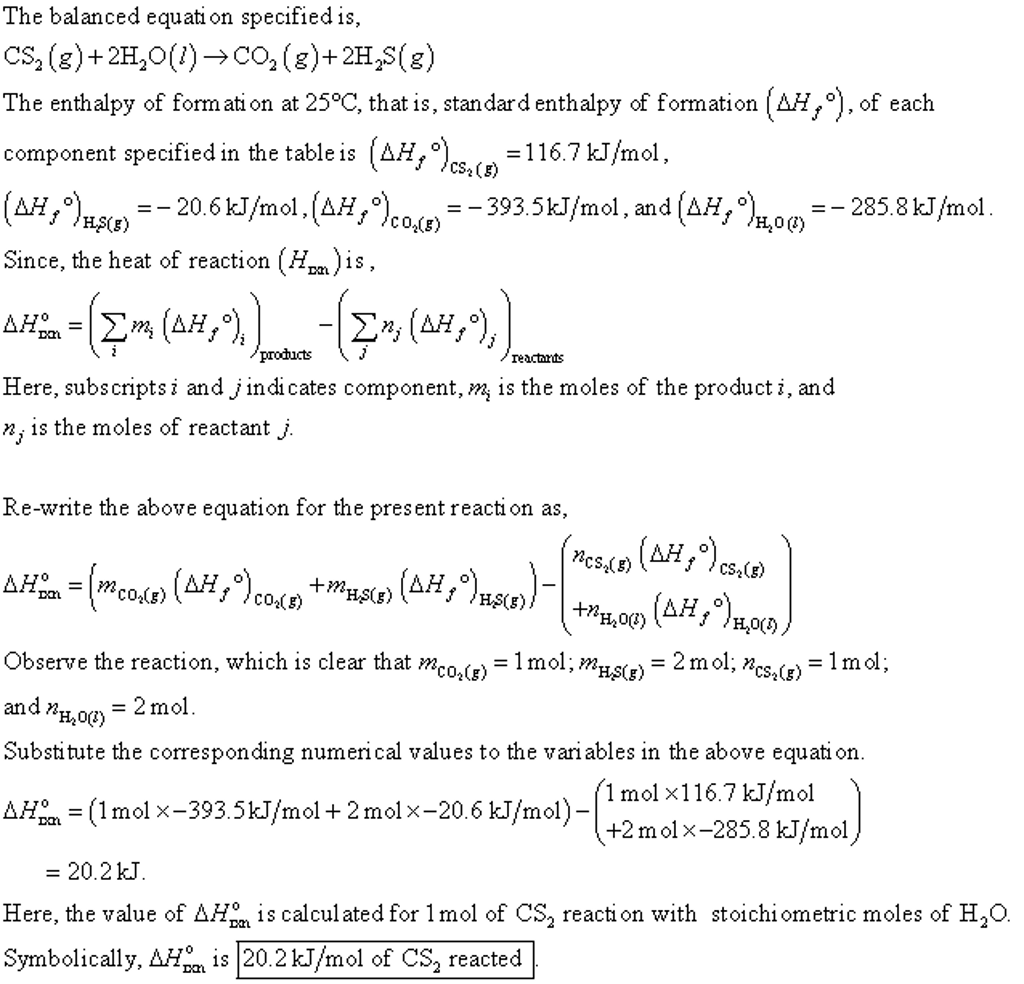# What is δh∘rxn for the following chemical reaction? cs2(g)+2h2o(l)→co2(g)+2h2s(g)

What is ΔH∘rxn for the following chemical reaction? H CS2(g)+2H2O(l)→CO2(g)+2H2S(g) You can use the following table of standard heats of formation
(ΔH∘f) to calculate the enthalpy of the given
reaction. H

Element/ Compound
Standard Heat of Formation (kJ/mol)
Element/ Compound
Standard Heat of Formation (kJ/mol)

H(g)
218
N(g)
473

H2(g)
0
O2(g)
0

H2O(l)
−285.8
O(g)
249

CS2(g)
116.7
H2S(g)
−20.60kJ

C(g)
71
CO2(g)
−393.5kJ

C(s)
0
HNO3(aq)
−206.6

Element/ Compound
Standard Heat of Formation (kJ/mol)
Element/ Compound
Standard Heat of Formation (kJ/mol)

H(g)
218
N(g)
473

H2(g)
0
O2(g)
0

H2O(l)
−285.8
O(g)
249

CS2(g)
116.7
H2S(g)
−20.60kJ

C(g)
71
CO2(g)
−393.5kJ

C(s)
0
HNO3(aq)
−206.6

Element/ Compound
Standard Heat of Formation (kJ/mol)
Element/ Compound
Standard Heat of Formation (kJ/mol)
Element/ Compound Element/ Compound Standard Heat of Formation (kJ/mol) Standard Heat of Formation (kJ/mol) Element/ Compound Element/ Compound Standard Heat of Formation (kJ/mol) Standard Heat of Formation (kJ/mol)
H(g)
218
N(g)
473
H(g) 218 N(g) 473
H2(g)
0
O2(g)
0
H2(g) 0 O2(g) 0
H2O(l)
−285.8
O(g)
249
H2O(l) −285.8 O(g) 249
CS2(g)
116.7
H2S(g)
−20.60kJ
CS2(g) 116.7 H2S(g) −20.60kJ
C(g)
71
CO2(g)
−393.5kJ
C(g) 71 CO2(g) −393.5kJ
C(s)
0
HNO3(aq)
−206.6
C(s) 0 HNO3(aq) −206.6 Express the standard enthalpy of reaction to three significant
figures and include the appropriate units.

The balanced equati on specified is, CS2 (g)+2H(CO2 (g)+2HS(g) The enthalpy of form ati on at 25°C, that is, standard enthalpy of formation [AH,°), of each component specified in the table is ΔΗ ,%(s)-116.7 kJ/mol (ΔΙ,еђна’)–20.6 kJ/mol, (AH,°) o (,) Since, the heat of reaction (Hpn) is –393.5kJ/mol, and AH, o ) – 285.8kJ/mol H^o (?) og) reacthrts Here, subscripts i and j indi cates component, m, is the moles of the producti, and n, is the moles of reactantj Re-write the above equation for the present reacti on as, Observe the reaction, which is clear that m 2mol, ns()mol CO2(g) Substitute the corresponding numerical values to the variables in the above equati on АНо,-(1 mol x-393.5 kJ/mol + 2 mol x-20.6 kJ/mol)- 1 molx116.7 kJ/mol +2molx-285.8 kJ/mol – 20.2kJ Here, the value of Ayon is calculated for 1 mol of CS2 reaction with stoichiometric moles of H2O Symbolically, s20.2kI/mol of CS2 reacted